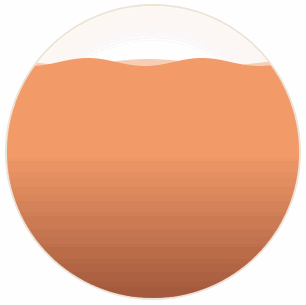# 水波图实现原理

23,808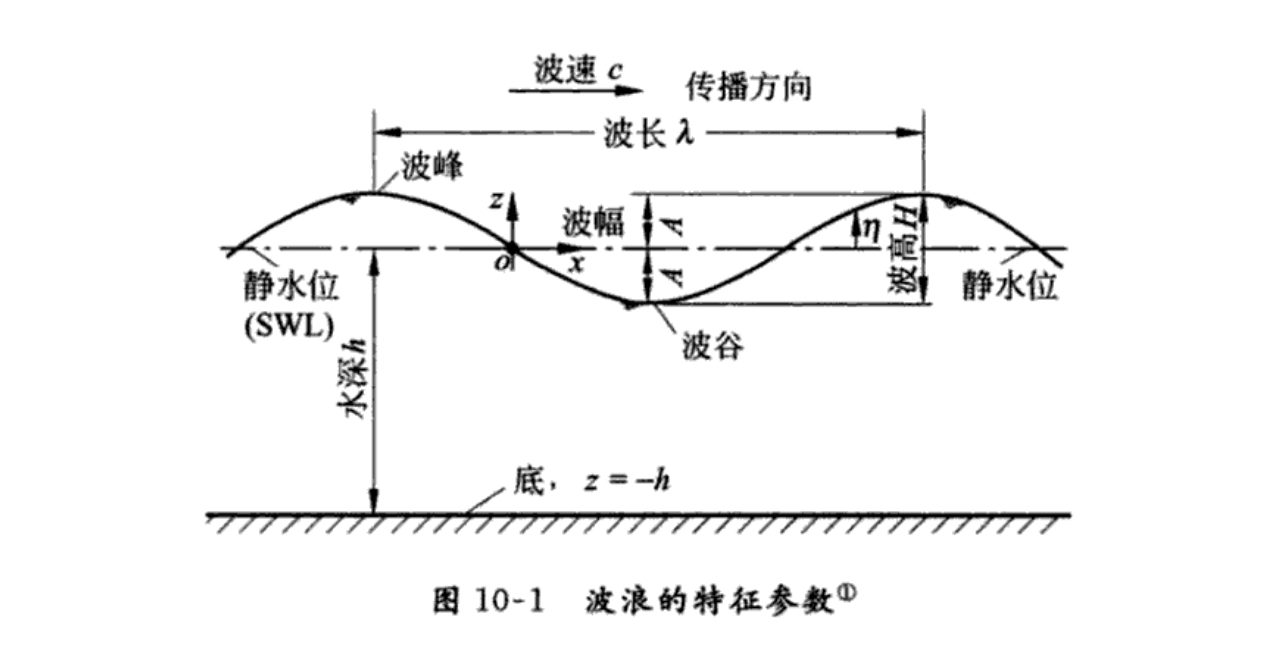## 正弦曲线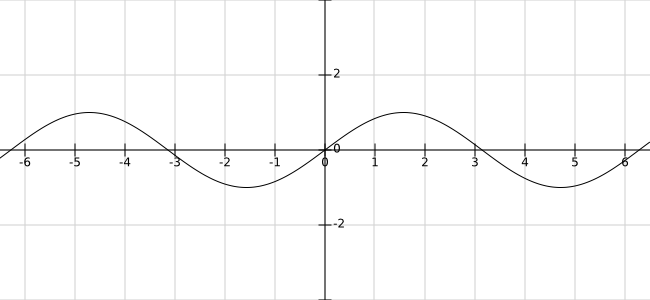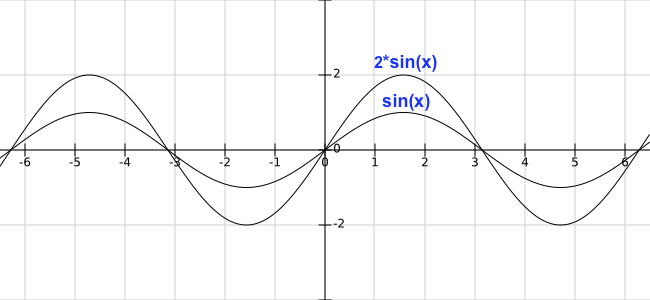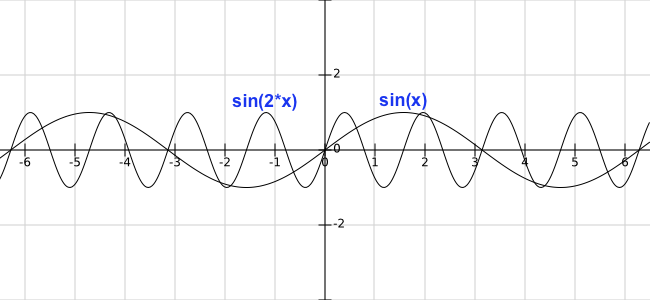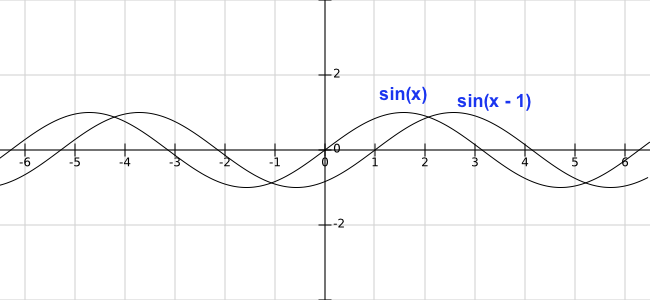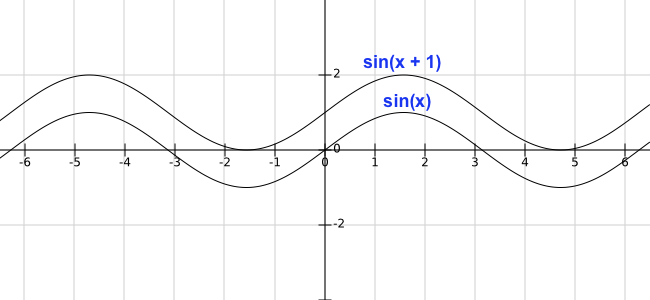• 振幅：控制波浪的高度
• 周期：控制波浪的宽度
• 相移：控制波浪的水平移动
• 垂直位移：控制水位的高度

## 曲线绘制

``````componentDidMount() {
const self = this;
const canvas = this.refs.canvas;
canvas.height = 500;
canvas.width = 500;
this.canvas = canvas;
this.canvasWidth = canvas.width;
this.canvasHeight = canvas.height;
const ctx = canvas.getContext('2d');
this.drawSin(ctx);
}
render() {
return (
<div className="content page">
<canvas ref="canvas"></canvas>
</div>
);
}
``````

``````drawSin(ctx) {
const points = [];
const canvasWidth = this.canvasWidth;
const canvasHeight = this.canvasHeight;
const startX = 0;
const waveWidth = 0.05; // 波浪宽度,数越小越宽
const waveHeight = 20; // 波浪高度,数越大越高
const xOffset = 0; // 水平位移

ctx.beginPath();
for (let x = startX; x < startX + canvasWidth; x += 20 / canvasWidth) {
const y = waveHeight * Math.sin((startX + x) * waveWidth + xOffset);
points.push([x, (canvasHeight / 2) + y]);
ctx.lineTo(x, (canvasHeight / 2) + y);
}
ctx.lineTo(canvasWidth, canvasHeight);
ctx.lineTo(startX, canvasHeight);
ctx.lineTo(points, points);
ctx.stroke();
}
``````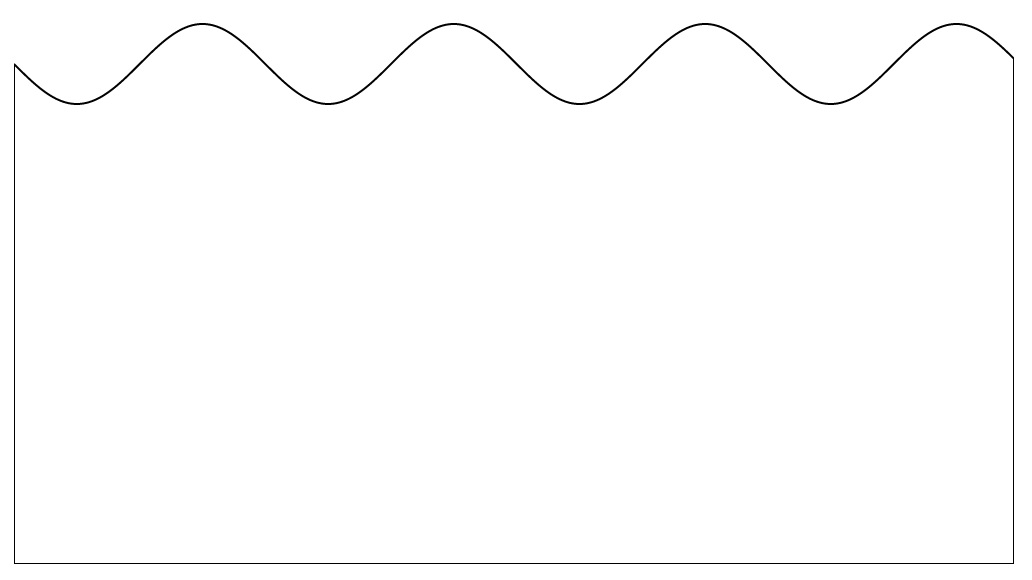``````componentDidMount() {
...
this.xOffset = 0;  // 初始偏移
this.speed = 0.1;  // 偏移速度
requestAnimationFrame(this.draw.bind(this, canvas));
}

draw() {
const canvas = this.canvas;
const ctx = canvas.getContext('2d');
ctx.clearRect(0, 0, canvas.width, canvas.height);
// 曲线绘制
this.drawSin(ctx, this.xOffset);
this.xOffset += this.speed;
requestAnimationFrame(this.draw.bind(this));
}

drawSin(ctx, xOffset = 0) {
...
}
``````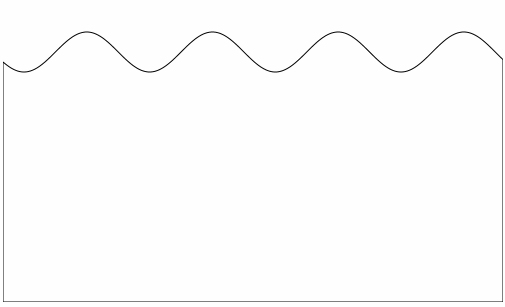## 球型绘制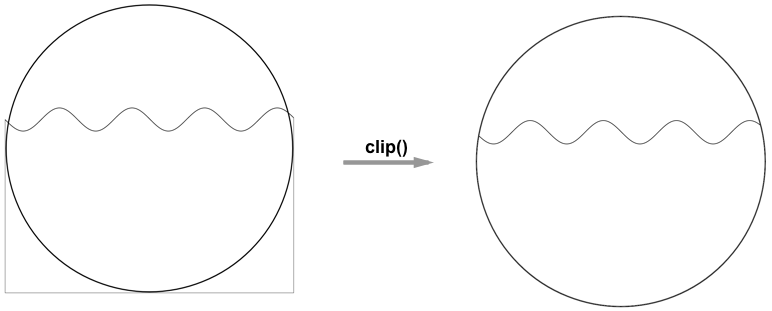``````draw() {
...
if (!this.isDrawCircle) {
this.drawCircle(ctx);
}
this.drawSin(ctx, this.xOffset);
this.xOffset += this.speed;
requestAnimationFrame(this.draw.bind(this));
}

drawCircle(ctx) {
const r = this.canvasWidth / 2;
const lineWidth = 5;
const cR = r - (lineWidth);
ctx.lineWidth = lineWidth;
ctx.beginPath();
ctx.arc(r, r, cR, 0, 2 * Math.PI);
ctx.stroke();
ctx.clip();
this.isDrawCircle = true;
}
``````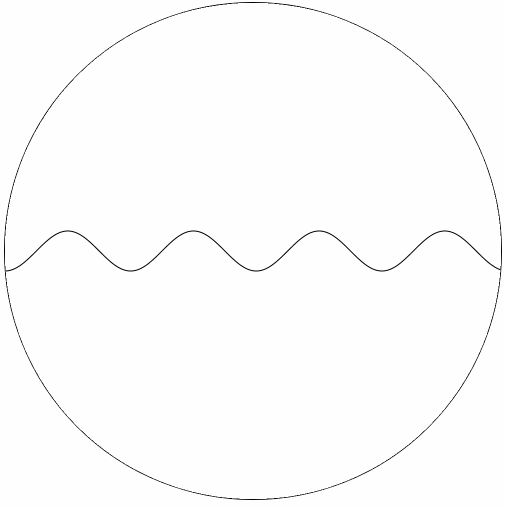## 水位控制

``````componentDidMount() {
...
this.xOffset = 0;
this.speed = 0.1;
// 水位数值
this.rangeValue = 0.6;
// 初始水位
this.nowRange = 0;
requestAnimationFrame(this.draw.bind(this, canvas));
}

draw() {
...
this.drawSin(ctx, this.xOffset, this.nowRange);
this.xOffset += this.speed;
if (this.nowRange < this.rangeValue) {
this.nowRange += 0.01;
}
requestAnimationFrame(this.draw.bind(this));
}

drawCircle(ctx) {
...
}

drawSin(ctx, xOffset = 0, nowRange = 0) {
...
for (let x = startX; x < startX + canvasWidth; x += 20 / canvasWidth) {
const y = waveHeight * Math.sin((startX + x) * waveWidth + xOffset);
points.push([x, (1 - nowRange) * canvasHeight + y]);
ctx.lineTo(x, (1 - nowRange) * canvasHeight + y);
}
...
}
``````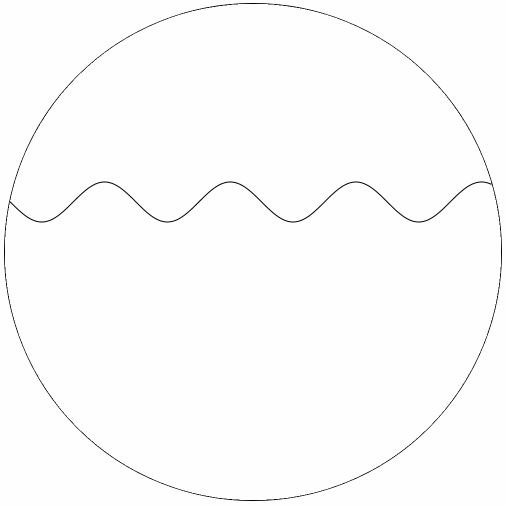## 最终效果